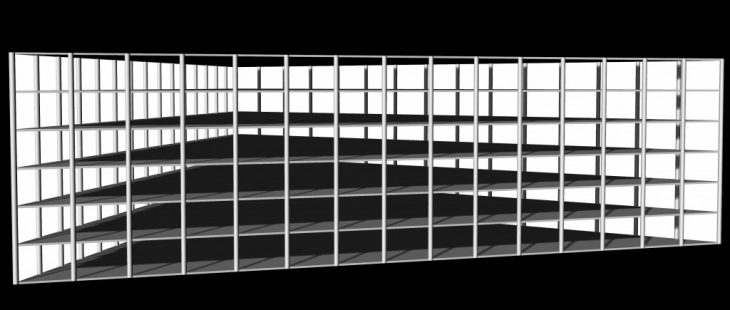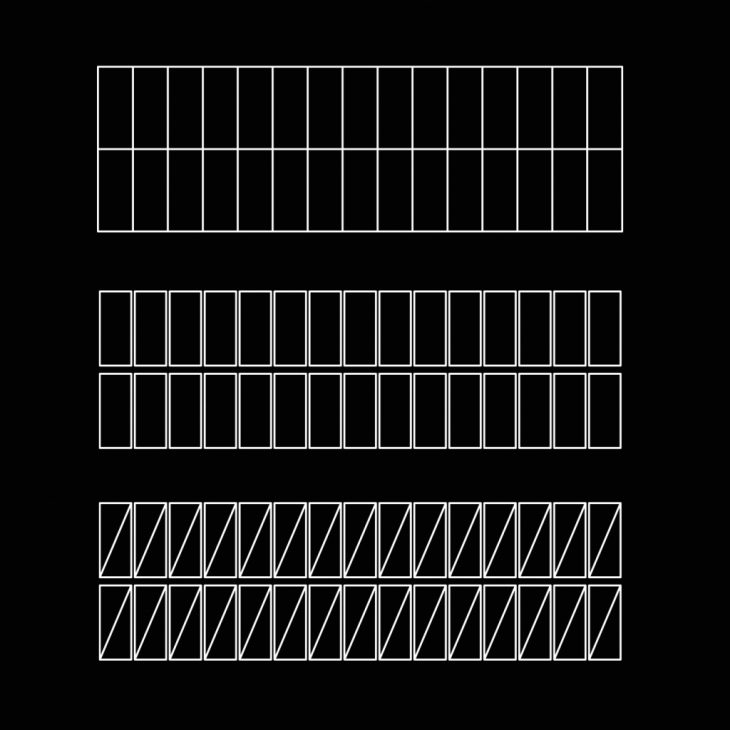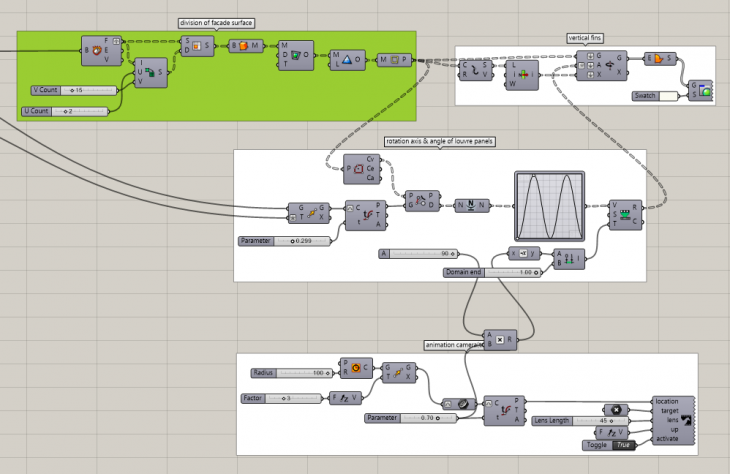MAA 01 2017-2018
S0.3 Computational design

Brief:

To create a dynamic animation mimicking a responsive facade system. The purpose of the animation is to visualize the solution proposed
underlining its reactive logic.

Project:Southern Denmark University, Kolding Campus

Architect: Henning Larsen

Process:

Formwork-Script for floors and columns

• A triangle is drawn for the base plan
• It is then moved in the y-axis using a ‘series’ to make the 6 levels of the building
the levels are extruded to floor width
• Each side of the triangular façade is divided into 15 equal parts
• A column is drawn from ground to roof the periphery is given a fixed glazing extrusion from ground to roofThe floor and column structure

• The three outer elevation facades are each subdivided into 30 parts, 15×2 on each facade
• Each part is then developed as a frame, deducting the surfaces of the columns
• Lastly, each part is split diagonally to achieve the desired triangulated panel• The center of each facade vertical fin is identified and points are pulled to the mid-level triangle polygon
• The distances from point to geometry are then normalised and put through a sine graph mapper for greater variation
• The panel frame polygon is exploded and the column edge is used as the rotation axis
• The triangular panels are then rotated using angle values obtained from remapping the sine graph values as angles